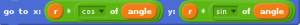## My Experience at the Workshop

NEW EMERGENCE

The word Workshop is very common in Auroville. Lots of workshops happen over the course of a year. But I never got the idea of attending any of the workshop(I also dont know why I didnt attend any and probably it never struck me).

Sanjeev then one day told us about a workshop called Stewardship for New Emergence (Monika Sharma’s workshop). He told us that the 1st stage of the workshop is for 9 days(I was like wow! 9 days!) and gave us a form to fill for the workshop. Just looking at the form made me feel that the workshop is going to be intense. Just filling up the form made me think a lot about myself.

The workshop was held over a period of 3 months(3 days a month).

We learnt about 40 tools in these 9 days. All the tools given in the workshop are very much related to everyone’s daily life. Every exercise given in the workshop would have somehow or the other happened in your life but I never have thought about how and why it happened, what I learnt is to differentiate the tools that was given.

For example, there was an exercise about ‘anger’. There are two types of anger(Destructive and Principled). This was the first time I heard about anger being differentiated into two types. All I knew about anger before was shouting and pouring emotions on someone(destructive anger). But after doing this exercise I came to know that sometimes even my anger was not destructive and I was Principled in my anger.

There was tool about ‘complaints’. We all listen to complaints made by others and get bored and agitated. But here i learnt to listen to the committments behind the complaints. It made me look at complaints made by other people on me in a dfiferent angle.

Like this i learnt a lot of new things that are needed for the growth of myself and the people around me. Just attending this 9 days(which was fully worth it) of workshop does not give someone a new life. It is important that I practice all the tools that I have learned.

Overall this workshop was totally a new and a different experience for me personally. I came to know more about myself and I think over these 9 days I have accomplished a New Emergence !!

## Wavelength

Wavelength

The wavelength of a sinusoidal wave is the spatial period of the wave—the distance over which the wave’s shape repeats. It is usually determined by considering the distance between consecutive corresponding points of the same phase.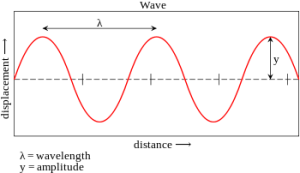The above figure shows the wavelength at a certain time period(the figure is freezed)

In linear media, any wave pattern can be described in terms of the independent propagation of sinusoidal components. The wavelength λ of a sinusoidal waveform traveling at constant speed v is given by

λ = v/f

where v is called the phase speed (magnitude of the phase velocity) of the wave and f is the wave’s frequency. In a dispersive medium, the phase speed itself depends upon the frequency of the wave, making the relationship between wavelength and frequency nonlinear.

In the case of electromagnetic radiation such as light in free space, the phase speed is the speed of light, about . Thus the wavelength of a 100 MHz electromagnetic (radio) wave is about: divided by 108 Hz = 3 meter.

For sound waves in air, the speed of sound is 343 m/s (at room temperature and atmospheric pressure). The wavelengths of sound frequencies audible to the human ear (20 Hz–20 kHz) are thus between approximately 17 m and 17 mm, respectively. Note that the wavelengths in audible sound are much longer than those in visible light.

Difference between Wavelength and Time Period :

There are two variables. One is time and other distance.

We can draw a curve with respect to time and say the vertical displacement at a particular point which shows the period and amplitude. It is time variant at particular point.

We can also draw a curve with respect to distance at a particular time which shows the wavelength and amplitude.

More animations about wavelength are given :

http://www.animations.physics.unsw.edu.au/jw/travelling_sine_wave.htm

## Circle Using Scratch

Polar Form of a Circle :
The polar coordinate system is a two-dimensional coordinate system in which each point on a plane is determined by a distance from a fixed point and an angle from a fixed direction. Keeping the radius as constant value(as the radius of the circle of the cant change), the angle keeps on varying until the circle is complete.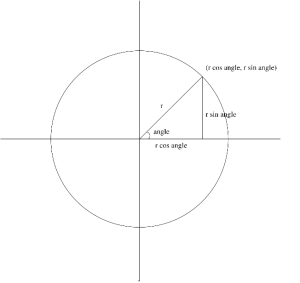Here, we have a circle with radius r.
Along the x- axis it is r cos angle and along the y-axis it is r sin angle.
x : r cos angle
y : r sin angle
Circle moved along the axis with radius r :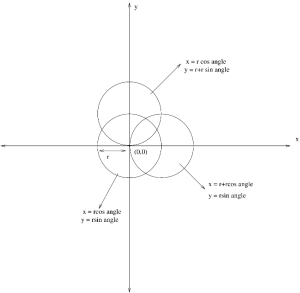Three circles are shown in the figure.
Each circle has a radius of r(defined by the user).
Center Circle :
The circle at the center has origin of (0,0) and by using the polar form method a simple circle can be drawn by defining the radius r and the angle.
x = r cos angle
y = r sin angle
Circle on the top :
The circle on the top is along y axis. The origin of this circle is at the edge of the center circle on the y axis. So,the equation of this circle is
x = r cos angle
y = r+r sin angle
Circle on the right :
The circle on the right is along x axis. The origin of this circle is at the edge of the center circle on the x axis. So,the equation of this circle is
x = r+r cos angle
y = r sin angle
Drawing a Circle in Scratch using the Trigonometric Formula :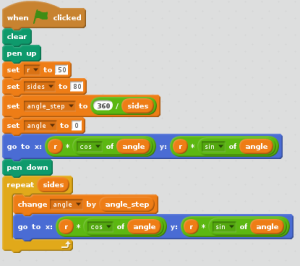There are number ways to draw a circle in Scratch. Lets have a look at how to draw a circle using the Trigonometric formula(Polar form).
Simple way to make a circle is to use the Motion Scripts and to use the Move keys and angle (360 degree) etc..
Create Variables :
Example ,